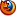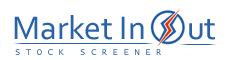Please enable JavaScript to view this page content properly Log In | Sign Up
 Trend Lines Fibonacci Levels DeMark's Approaches Support & Resistance Pivot Points Previous High/Low Linear Regression
 Fibonacci Levels Action Price Touched 0.23 Fibonacci Support Level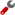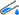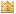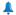Price Touched 0.23 Fibonacci Support Level and ReversedPrice Touched 0.38 Fibonacci Support LevelPrice Touched 0.38 Fibonacci Support Level and ReversedPrice Touched 0.50 Fibonacci Support LevelPrice Touched 0.50 Fibonacci Support Level and ReversedPrice Touched 0.62 Fibonacci Support LevelPrice Touched 0.62 Fibonacci Support Level and ReversedPrice Touched 0.78 Fibonacci Support LevelPrice Touched 0.78 Fibonacci Support Level and ReversedPrice Touched 0.23 Fibonacci Resistance LevelPrice Touched 0.23 Fibonacci Resistance Level and ReversedPrice Touched 0.38 Fibonacci Resistance LevelPrice Touched 0.38 Fibonacci Resistance Level and ReversedPrice Touched 0.50 Fibonacci Resistance LevelPrice Touched 0.50 Fibonacci Resistance Level and ReversedPrice Touched 0.62 Fibonacci Resistance LevelPrice Touched 0.62 Fibonacci Resistance Level and ReversedPrice Touched 0.78 Fibonacci Resistance LevelPrice Touched 0.78 Fibonacci Resistance Level and Reversed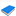Fibonacci Studies - Technical Analysis from A to Z Leonardo Fibonacci was a mathematician who was born in Italy around the year 1170. It is believed that Mr. Fibonacci discovered the relationship of what are now referred to as Fibonacci numbers while studying the Great Pyramid of Gizeh in Egypt. Fibonacci numbers are a sequence of numbers in which each successive number is the sum of the two previous numbers. These numbers possess an intriguing number of interrelationships, such as the fact that any given number is approximately 1.618 times the preceding number and any given number is approximately 0.618 times the following number. There are four popular Fibonacci studies: arcs, fans, retracements, and time zones.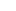Best viewed in